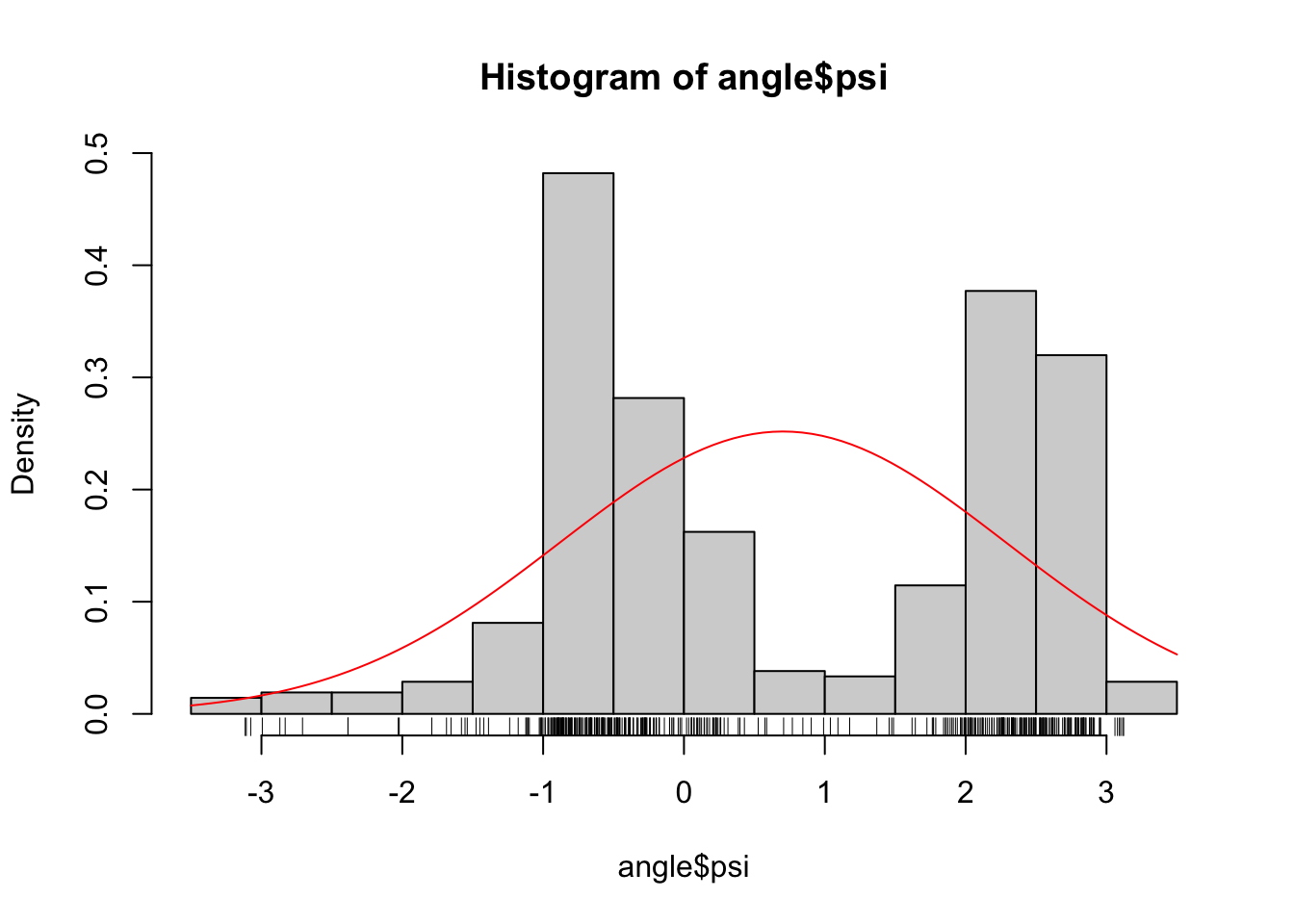## 2.1 Univariate density estimation

Recall the data on $$\phi$$- and $$\psi$$-angles in polypeptide backbone structures, as considered in Section 1.1.1.Figure 2.1: Histograms equipped with a rug plot of the distribution of $$\phi$$-angles (left) and $$\psi$$-angles (right) of the peptide planes in the protein human protein 1HMP.

We will in this section treat methods for smooth density estimation for univariate data such as data on either the $$\phi$$- or the $$\psi$$-angle.

We let $$f_0$$ denote the unknown density that we want to estimate. That is, we imagine that the data points $$x_1, \ldots, x_n$$ are all observations drawn from the probability measure with density $$f_0$$ w.r.t. Lebesgue measure on $$\mathbb{R}$$.

Suppose first that $$f_0$$ belongs to a parametrized statistical model $$(f_{\theta})_{\theta}$$, where $$f_{\theta}$$ is a density w.r.t. Lebesgue measure on $$\mathbb{R}$$. If $$\hat{\theta}$$ is an estimate of the parameter, $$f_{\hat{\theta}}$$ is an estimate of the unknown density $$f_0$$. For a parametric family we can always try to use the MLE $\hat{\theta} = \text{arg max}_{\theta} \sum_{j=1}^n \log f_{\theta}(x_j)$ as an estimate of $$\theta$$. Likewise, we might compute the empirical mean and variance for the data and plug those numbers into the density for the Gaussian distribution, and in this way obtain a Gaussian density estimate of $$f_0$$.

psi_mean <- mean(psi)
psi_sd <- sd(psi)
hist(psi, prob = TRUE)
rug(psi)
curve(dnorm(x, psi_mean, psi_sd), add = TRUE, col = "red")Figure 2.2: Gaussian density (red) fitted to the $$\psi$$-angles.

As Figure 2.2 shows, if we fit a Gaussian distribution to the $$\psi$$-angle data we get a density estimate that clearly does not match the histogram. The Gaussian density matches the data on the first and second moments, but the histogram shows a clear bimodality that the Gaussian distribution by definition cannot match. Thus we need a more flexible parametric model than the Gaussian if we want to fit a density to this data set.

In nonparametric density estimating we want to estimate the target density, $$f_0$$, without assuming that it belongs to a particular parametrized family of densities.